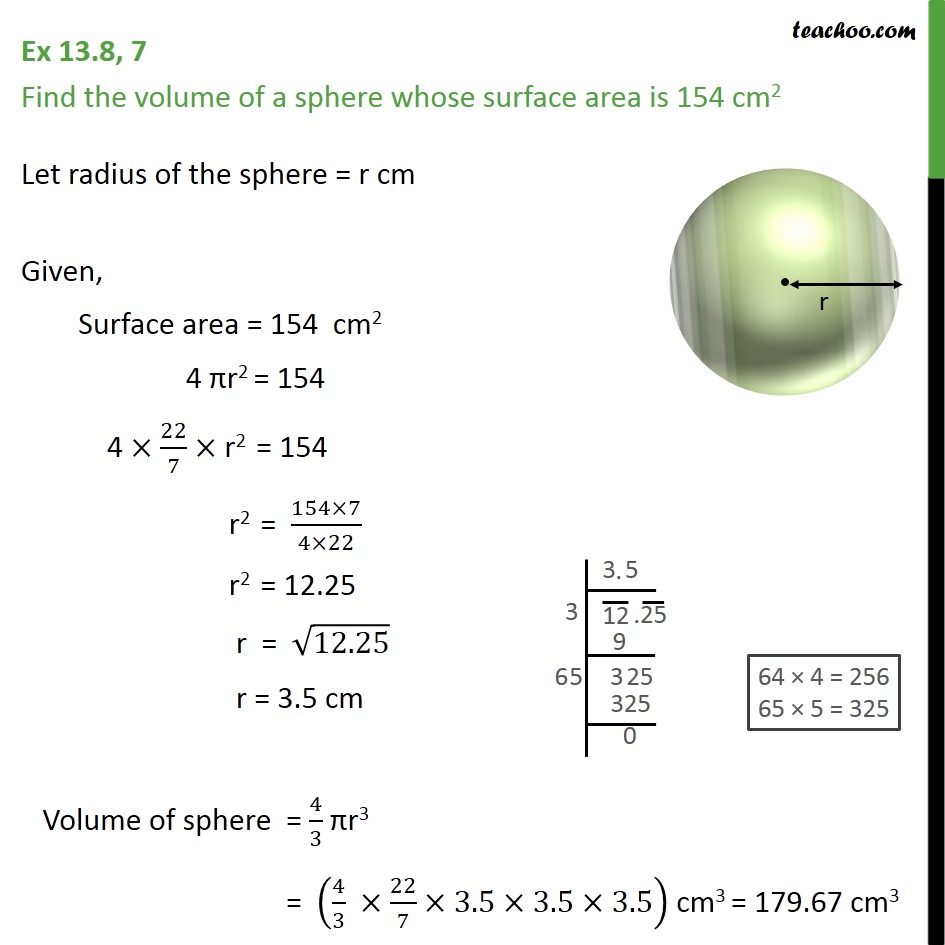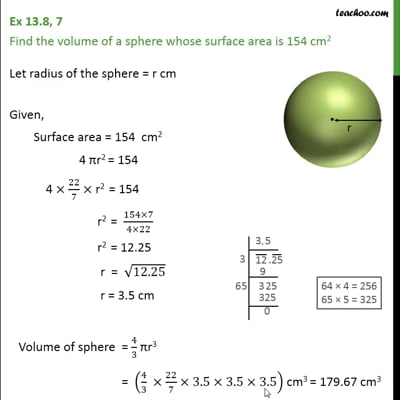Ex 13.8

Chapter 13 Class 9 Surface Areas and Volumes
Serial order wiseThis video is only available for Teachoo black users

Solve all your doubts with Teachoo Black (new monthly pack available now!)

### Transcript

Ex 13.8, 7 Find the volume of a sphere whose surface area is 154 cm2 Let radius of the sphere = r cm Given, Surface area = 154 cm2 4 r2 = 154 4 22/7 r2 = 154 r2 = (154 7)/(4 22) r2 = 12.25 r = 12.25 r = 3.5 cm Volume of sphere = 4/3 r3 = (4/3 22/7 3.5 3.5 3.5) cm3 = 179.67 cm3